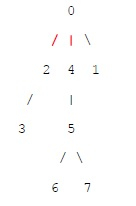# Maximum edge removal from tree to make even forest in C++

## Problem statement

Given an undirected tree which has even number of vertices, we need to remove the maximum number of edges from this tree such that each connected component of the resultant forest has an even number of vertices.

## ExampleIn above shown tree, we can remove at max 2 edges 0-2 and 0-4 shown in red such that each connected component will have even number of vertices.

## Algorithm

• Do DFS from any starting node as tree is connected
• Initialize count of nodes in subtree rooted under current node as 0
• Do following recursively for every subtree of current node −
• If size of current subtree is even, increment result by 1 as we can disconnect the subtree
• Else add count of nodes in current subtree to current count

## Example

Let us now see an example −

Live Demo

#include <bits/stdc++.h>
using namespace std;
int dfs(vector<int> g[], int u, bool visit[], int& res) {
visit[u] = true;
int currComponentNode = 0;
for (int i = 0; i < g[u].size(); i++) {
int v = g[u][i];
if (!visit[v]) {
int subtreeNodeCount = dfs(g, v, visit, res);
if (subtreeNodeCount % 2 == 0)
res++;
else
currComponentNode += subtreeNodeCount;
}
}
return (currComponentNode + 1);
}
int maxEdgeRemovalToMakeForestEven(vector<int> g[], int N) {
bool visit[N + 1];
for (int i = 0; i <= N; i++)
visit[i] = false;
int res = 0;
dfs(g, 0, visit, res);
return res;
}
void addEdge(vector<int> g[], int u, int v) {
g[u].push_back(v);
g[v].push_back(u);
}
int main() {
int edges[] = {{0, 2}, {0, 1}, {0, 4}, {2, 3}, {4, 5}, {5, 6}, {5, 7} };
int N = sizeof(edges)/sizeof(edges); vector<int> g[N + 1];
for (int i = 0; i < N; i++)
}
Answer = 2